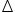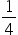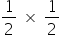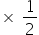Mathematics
Easy

Question

# In the given triangular pyramid, ABCP, YZ is the midsegment ofAPC, XZ is the midsegment ofABC, XY is the midsegment ofABP. Which of the following statement is true?

## Area ofBPC =Area ofXYZArea of ∆ XYZ = 2 Area of ∆ BPCArea of ∆ABC = Area of ∆ABPAll the aboveHint:

## The correct answer is: Area ofBPC =Area ofXYZ

### Area of ∆BPC =height of ∆XYZbase of ∆XYZ=Area of ∆XYZ

The midpoint theorem refers to the midpoint of the line segment. It defines the coordinate points of the midpoint of the line segment and can be found by taking the average of the coordinates of the given endpoints.

### Related Questions to study#### With Turito Foundation.#### Get an Expert Advice From Turito.